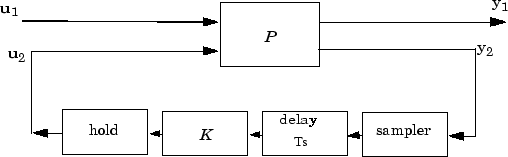# sdhinfsyn

Compute H controller for sampled-data system

## Syntax

```[K,GAM]=sdhinfsyn(P,NMEAS,NCON)
[K,GAM]=sdhinfsyn(P,NMEAS,NCON, KEY1,VALUE1,KEY2,VALUE2,...)
```

## Description

`sdhinfsyn` controls a continuous-time LTI system P with a discrete-time controller `K`. The continuous-time LTI plant `P` has a state-space realization partitioned as follows:

`$P=\left[\begin{array}{ccc}A& {B}_{1}& {B}_{2}\\ {C}_{1}& 0& 0\\ {C}_{2}& 0& 0\end{array}\right]$`

where the continuous-time disturbance inputs enter through B1, the outputs from the controller are held constant between sampling instants and enter through B2, the continuous-time errors (to be kept small) correspond to the C1 partition, and the output measurements that are sampled by the controller correspond to the C2 partition. B2 has column size `ncon` and C2 has row size `nmeas`. Note that the D matrix must be zero.

`sdhinfsyn` synthesizes a discrete-time LTI controller `K` to achieve a given norm (if possible) or find the minimum possible norm to within tolerance `TOLGAM`.Similar to `hinfsyn`, the function `sdhinfsyn` employs a γ iteration. Given a high and low value of γ, `GMAX` and `GMIN`, the bisection method is used to iterate on the value of γ in an effort to approach the optimal H control design. If `GMAX` = `GMIN`, only one γ value is tested. The stopping criterion for the bisection algorithm requires that the relative difference between the last γ value that failed and the last γ value that passed be less than `TOLGAM`.

Input arguments

 `P` LTI plant `NMEAS` Number of measurements output to controller `NCON` Number of control inputs

Optional input arguments (`KEY, VALUE`) pairs are similar to `hinfsyn`, but with additional `KEY` values `'Ts'` and `'DELAY'`.

KEY

VALUE

Meaning

`'GMAX'`

real

Initial upper bound on GAM (default=`Inf`)

`'GMIN'`

real

Initial lower bound on GAM (default=0)

`'TOLGAM'`

real

Relative error tolerance for GAM (default=.01)

`'Ts'`

real

(Default=1) sample time of the controller to be designed

`'DELAY'`

integer

(Default=0) a nonnegative integer giving the number of sample periods delay for the control computation

`'DISPLAY'`

`'off'`

`'on'`

(Default) no command window display, or the command window displays synthesis progress information

Output arguments

 `K` H∞ controller `GAM` Final γ value of H∞ cost achieved

## Algorithms

`sdhinfsyn` uses a variation of the formulas described in the Bamieh and Pearson paper  to obtain an equivalent discrete-time system. (This is done to improve the numerical conditioning of the algorithms.) A preliminary step is to determine whether the norm of the continuous-time system over one sampling period without control is less than the given γ-value. This requires a search and is computationally a relatively expensive step.

## References

 Bamieh, B.A., and J.B. Pearson, “A General Framework for Linear Periodic Systems with Applications to Sampled-Data Control,” IEEE Transactions on Automatic Control, Vol. AC–37, 1992, pp. 418-435.

## Version History

Introduced before R2006a